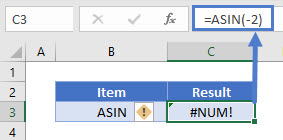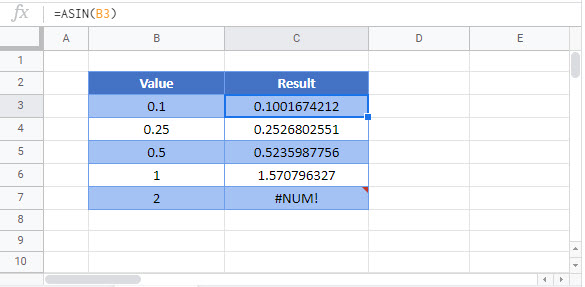### ASIN Function Examples – Excel & Google Sheets

This tutorial demonstrates how to use the Excel ASIN Function returns the inverse sine of a number in radians.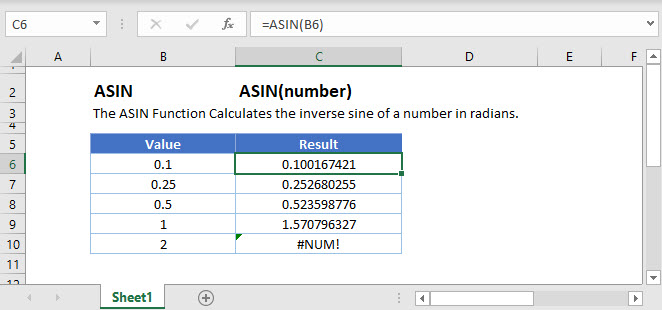## ASIN Function Overview

The ASIN Function returns the inverse sine of a number in radians.

To use the ASIN Excel Worksheet Function, select a cell and type: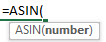(Notice how the formula inputs appear)

### ASIN Function Syntax and Inputs:

number – The value to get the inverse sine of. The number must be between -1 and 1 inclusive.

AutoMacro - VBA Code Generator

## ASIN Function

The ASIN Function returns the inverse sine of a number in radians.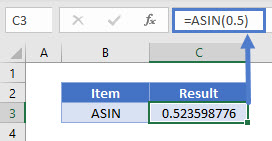## ASIN & PI Function

The ASIN Function returns the inverse sine of a number in radians. To convert it into degrees, multiply by 180 and divide by the PI Function.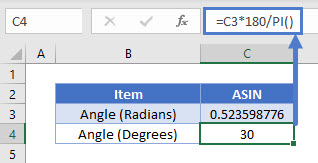## ASIN & DEGREES Function

Alternatively, the DEGREES Function can be directly used to convert the radians output into degrees.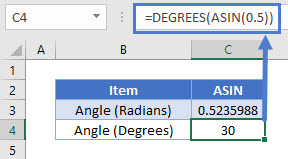## ASIN Function – Out Of Range

The ASIN Function will return a value if the input lies in the range of -1 to 1. Otherwise the ASIN Function will return an error.